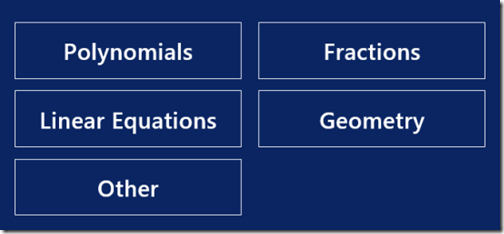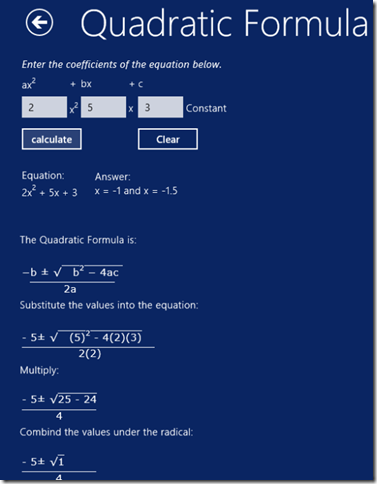Editor Ratings:
User Ratings:
[Total: 1 Average: 5]

Math Solver is a free app for Windows 8 which solves Math problems in a single click. The app lets you solve math problems in following categories: Polynomials, Fractions, Linear Equations, Geometry, and Other. Each category contains different sub-categories related to topics of Math. For example, Polynomials contains different sub-categories like: Quadratic Formula, Vertex, Factoring, Rational Root Test, and Synthetic design. Each sub-category contains the general equation of the same.

The Other category lets you solve problems like: Ohms law, Unit conversion, Factoring, etc.. This app not only shows answers, but explains the steps/methods used to solve the problem as well.## Key Features of Math Solver App For Windows 8:

• Easy to use interface.
• Covers many topics of Math like: Polynomials, Fractions, Linear Equations, Geometry, and many more.
• Provides other related categories like: Ohms law, Unit conversion, Factoring, etc..
• Contains general equations of all the topics.
• You just need to enter values to get the solution.
• Solves any supported problem quickly

You can also try other similar app reviewed by us earlier: SpeQ Mathematics.

## How to Use Math Solver App For Windows 8?

You can get free Math Solver App from Windows 8 Store, or by clicking on the link given at the end of this review.

After launching the app, you will find five categories on the home screen: Polynomials, Fractions, Linear Equations, Geometry, and Other.• Polynomials contains different topics of Polynomials: Quadratic Formula, Vertex, Factoring, Rational Root Test, and Synthetic Division.
• Fractions contains different sub-topics: Fraction Simplifier, Combining Fractions, and Decimal to fractions.
• Linear Equations provides sub-topics: Slope of a Line, Equation of a Line, and Distance Formula.
• Geometry has different sub-topics like: Right Triangles, Non-Right triangles, Circles, Rectangular Prism, Cone, Sphere, Cylinder, and Pyramid.
• Other contains different topics like: Unit conversion, Radical Simplifier, Factorials, Prime Factors, Percent, and Ohms law.

Choose any topic as per your Math problem, and then type numerical values required to solve the problem. You will get the answer with solution in no time. For example: If you have a problem related to Quadratic formula, then click on Polynomials and then on Quadratic formula. Type the coefficient in the relevant box. Click calculate to get solution.Similarly, you can solve other Math problems as well.

One drawback of the app is that neither you can save the solution of the program in your local system nor can you share it online.

## Conclusion:

Math solver is a very useful app for solving Math problems. It covers a wide variety of Math areas. Most importantly, it shows all the steps involved in solving a problem, so that you also get an understanding of it.